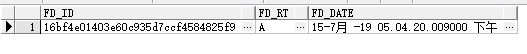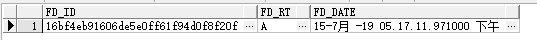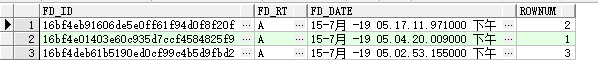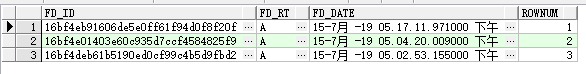## oracle 获取第一条数据

``````SELECT * FROM tableName where fd_rt = 'A'
--and rownum=1
ORDER BY fd_date DESC ``````

``````SELECT * FROM tableName where fd_rt = 'A'
and rownum=1
ORDER BY fd_date DESC ````````````SELECT t.* from
(
SELECT * FROM tableName where fd_rt = 'A' ORDER BY fd_date DESC
) t WHERE rownum = 1``````rownum作为伪列实际上查询结果为：

``````SELECT tableName .*,rownum FROM tableName where fd_rt = 'A'
--and rownum=1
ORDER BY fd_date DESC ````````````SELECT t.*,rownum from
(
SELECT * FROM tableName where fd_rt = 'A' ORDER BY fd_date DESC
) t``````